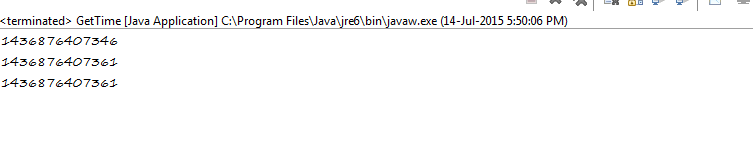# Code In Action

## 3 Ways to Get the Current Time In MilliSeconds In Java?

You can get the current time in milli seconds in 3 ways in java.

1. By Using Date class:

The Date class has a method named getTime(). It returns the number of milliseconds since January 1, 1970, 00:00:00 GMT represented by this Date object. The code for this is
```     Date d = new Date();
System.out.println(d.getTime());
```

2.By Using Calendar class:

The Calendar class has getTimeInMillis() for this purpose. It returns this Calendar's time value in milliseconds.Below is the code for this.
```    Calendar c = Calendar.getInstance();
System.out.println(c.getTimeInMillis());
```

3.By Using System class:

The currentTimeMillis() method can be used to get the time in milli seconds. It is a static method in System class. It returns the difference, measured in milliseconds, between the current time and midnight, January 1, 1970 UTC. The code is
`System.out.println(System.currentTimeMillis());`

Unlike Date and Calendar classes, which require an object to be created, in this approach you can just call the currentTimeMillis() method, which reduces cost of creation of objects.

The complete code is below,
```package com.speakingcs.calendar;

import java.util.Calendar;
import java.util.Date;

public class GetTime {

public static void main(String[] args) {

// by using Date class
Date d = new Date();
System.out.println(d.getTime());

// by using Calendar class
Calendar c = Calendar.getInstance();
System.out.println(c.getTimeInMillis());

// by using System class. which removes overhead of creating Date
// and Calendar objects.
System.out.println(System.currentTimeMillis());

}
}
```

The output is: